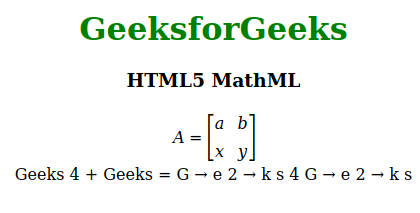Open in App
Not now

# HTML5 MathML Complete Reference

• Last Updated : 23 Jan, 2023

The MathML comes in HTML5 the current MathML version is 3 it was introduced in the year 2015. The MathML stands for Mathematics Markup Language. It is used to represent mathematical equations or expressions in web browsers like other HTML elements. The MathML is used to describe mathematics as a basis for the machine to machine communication, it is intended to be handled by specialized authoring tools such as equation editors and it is meaningful to other applications also.

Example:

## html

 `` `<``html``>`   `<``head``>` `    ``<``title``>HTML5 MathML` ``   `<``body` `style``=``"text-align:center;"``>`   `    ``<``h1` `style``=``"color:green"``>` `        ``GeeksforGeeks` `    ```   `    ``<``h3``>HTML5 MathML`   `    ``` `    ``<``math``>`   `        ``` `        ``<``mrow``>` `            ``<``mi``>A` `            ``<``mo``>=`   `            ``<``mfenced` `open``=``"["` `close``=``"]"``>`   `                ``<``mtable``>` `                    ``<``mtr``>` `                        ``<``mtd``>` `                            ``<``mi``>a` `                        ``` `                        ``<``mtd``>` `                            ``<``mi``>b` `                        ``` `                    ```   `                    ``<``mtr``>` `                        ``<``mtd``>` `                            ``<``mi``>x` `                        ``` `                        ``<``mtd``>` `                            ``<``mi``>y` `                        ``` `                    ```   `                ```   `            ``` `        ```   `        ``` `        ``<``br``><``br``>` `        ``<``msub``>` `            ``<``mi``>Geeks` `            ``<``mn``>4` `        ``` `        ``<``mo``>+` `        ``<``mn``>Geeks` `        ``<``mo``>=` `        ``<``msub``>` `            ``<``mi``>G` `        ``` `        ``<``mo``>→` `        ``<``msub``>` `            ``<``mi``>e` `            ``<``mn``>2` `        ``` `        ``<``mo``>→` `        ``<``mi``>k` `        ``<``mi``>s` `        ``<``mn``>4` `        ``` `        ``<``msub``>` `            ``<``mi``>G` `        ``` `        ``<``mo``>→` `        ``<``msub``>` `            ``<``mi``>e` `            ``<``mn``>2` `        ``` `        ``<``mo``>→` `        ``<``mi``>k` `        ``<``mi``>s` `    ``` ``   ``

Output:My Personal Notes arrow_drop_up
Related Articles